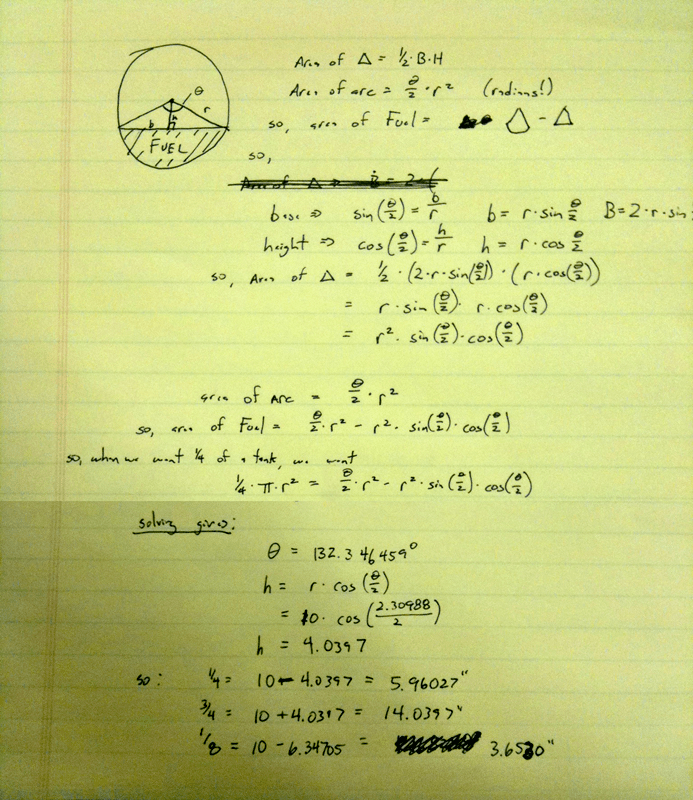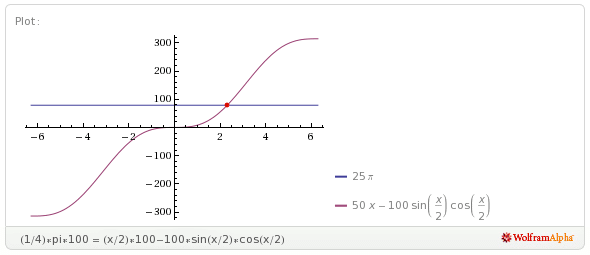Cartalk conundrum

# Cartalk conundrum

The guys from Car Talk have weekly puzzlers, but this question wasn’t a puzzler, but this came from a truck driver who called in.  He said (basically):

“I have big cylindrical tanks on my truck that lays sideways under the foot step.  Problem is that my gas gauge is broken.  I have a stick that I can put in vertically, so if the gas is at the 20″ mark on the stick, it’s full.  If the gas reaches the 10″ mark on the stick, the tank is clearly half full.  Where should I put the 1/4 and 3/4 marks on the stick?”

First you’d think they should be at 5″ and 15″, but that’s not right because the tank is round, which means the bottom and tops have less ‘volume’ per inch of height.   Then you think this is a problem is an integration problem – which it can be – but the integration becomes extremely hairy.  Then, you find you can back up and use a geometric method (and when you can’t reduce anymore) use a numerical method to solve it.  So let’s get started!

We see that needing the actual volume of the cylinder is unimportant since you can solve this problem with just the cross-section – which is a circle.  What you want is a circle with a chord across it in which the volume between the chord and the outer wall is 1/4 the capacity of the circle.  So, you draw a diagram, and get started!Unfortunately, you see that the equation becomes very difficult to solve analytically – and one must resort to numerical methods to get an actual solution.  I used the Mathematica online site, but you could easily use the Newton-Raphson method as well.  Whatever way you use, you find that he should mark the 1/4 tank line 5.96027 inches from the bottom of the stick.  3/4 and 1/8th values are also shown.

The value of this equation can quickly be used to calculate 1/8, 1/16, and all other desired fill marks by simply changing the 1/4 * pi * r^2 line whichever fraction you’d like. In fact, you can graph it to get any value:Ignoring negative volumes, you see that the tank’s volume compared to it’s theta (roughly equivalent to height) forms a S curve, so that you can see that the height changes more rapidly w.r.t. volume when close to full/empty than in the middle – just like we’d expect.

So, that’s your answer.  Turns out, others have solved this since it’s a common problem with all kinds of other tanks (fuel oil, gas stations, etc).  Here and here are other solutions that verify the same process and confirm that the final equation is unsolvable analytically.

Another person pointed out that most semi’s have TWO tanks – one on each side – which are connected by a balancing flow connector.  So both tanks fill and empty evenly.  Even though this seems to mess up the problem, it actually does not.  In order to represent that situation, you simply multiply both sides by two (two tanks, two times the target volume) – which cancel each other out.  You could have ANY number of tanks connected like this and the answer is the same.

It also doesn’t matter how long the tank is either (so long as the tanks are the same size if you have more than one).  Finally, the theta angle you calculate doesn’t even depend on what radius of the tank!  So if you calculate the thetas for all the fill points, then you can calculate the 1/4 mark on ANY size tank.  Pretty nifty huh.

This site uses Akismet to reduce spam. Learn how your comment data is processed.